##### Math For Real Life For DummiesSometimes you come across situations where you need to operate on real and imaginary numbers together, so you want to write both numbers as complex numbers in order to be able to add, subtract, multiply, or divide them.

Consider the following three types of complex numbers:

• A real number as a complex number: 3 + 0i

Notice that the imaginary part of the expression is 0.

• An imaginary number as a complex number: 0 + 2i

Notice that the real portion of the expression is 0.

• A complex number with both a real and an imaginary part: 1 + 4i

This number can't be described as solely real or solely imaginary — hence the term complex.

You can manipulate complex numbers arithmetically just like real numbers to carry out operations. You just have to be careful to keep all the i's straight. You can't combine real parts with imaginary parts by using addition or subtraction, because they're not like terms, so you have to keep them separate. Also, when multiplying complex numbers, the product of two imaginary numbers is a real number; the product of a real and an imaginary number is still imaginary; and the product of two real numbers is real. Many people get confused with this topic.

The following list presents the possible operations involving complex numbers.

• To add and subtract complex numbers: Simply combine like terms. For example, (3 – 2i) – (2 – 6i) = 3 – 2i – 2 + 6i = 1 + 4i.

• To multiply when a complex number is involved, use one of three different methods, based on the situation:

• To multiply a complex number by a real number: Just distribute the real number to both the real and imaginary part of the complex number. For example, here's how you handle a scalar (a constant) multiplying a complex number in parentheses: 2(3 + 2i) = 6 + 4i.

• To multiply a complex number by an imaginary number: First, realize that the real part of the complex number becomes imaginary and that the imaginary part becomes real. When you express your final answer, however, you still express the real part first followed by the imaginary part, in the form A + Bi.

For example, here's how 2i multiplies into the same parenthetical number: 2i(3 + 2i) = 6i + 4i2. Note: You define i as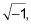so that i2 = –1! Therefore, you really have 6i + 4(–1), so your answer becomes –4 + 6i.

• To multiply two complex numbers: Simply follow the FOIL process (First, Outer, Inner, Last). For example, (3 – 2i)(9 + 4i) = 27 + 12i – 18i – 8i2, which is the same as 27 – 6i – 8(–1), or 35 – 6i.

• To divide complex numbers: Multiply both the numerator and the denominator by the conjugate of the denominator, FOIL the numerator and denominator separately, and then combine like terms. This process is necessary because the imaginary part in the denominator is really a square root (of –1, remember?), and the denominator of the fraction must not contain an imaginary part.

For example, say you're asked to divide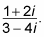The complex conjugate of 3 – 4i is 3 + 4i. Follow these steps to finish the problem:

1. Multiply the numerator and the denominator by the conjugate.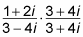2. FOIL the numerator.

You go with (1 + 2i)(3 + 4i) = 3 + 4i + 6i + 8i2, which simplifies to (3 – 8) + (4i + 6i), or –5 + 10i.

3. FOIL the denominator.

You have (3 – 4i)(3 + 4i), which FOILs to 9 + 12i – 12i – 16i2. Because i2 = –1 and 12i – 12i = 0, you're left with the real number 9 + 16 = 25 in the denominator (which is why you multiply by 3 + 4i in the first place).

4. Rewrite the numerator and the denominator.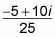This answer still isn't in the right form for a complex number, however.

5. Separate and divide both parts by the constant denominator.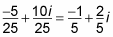Notice that the answer is finally in the form A + Bi.